# Congruence of Triangles – Mathematical reasoning

Example 1. Which of the following statemants is INCORRECT?

A. Two triangles having same area are congruent.

B. If two sides and one angle of a triangle are equal to the corresponding two sides and the angle of another triangle, then the two triangles are congruent.

C. If the hypotenuse of one right angled triangle is equal to the hypotencuse of another right angled triangle, then the triangles are congruent.

D. All of these.

Solution:

D

Example 2. In $$\Delta ABC,\text{ }AB=AC$$ and AD is perpendicular bisector of BC. The property by which  $$\Delta ADB$$ is not congruent to  $$\Delta ADC$$ is­­­­­­­­­ ­­­­­­­­_______.

A. SAS property

B. SSS property

C. RHS property

D. AAA property.

Solution:

D

Example 3. In the given figure, $$PA\bot AB,\text{ }QB\bot AB$$ and  $$\Delta OAP\cong \Delta OBQ$$, then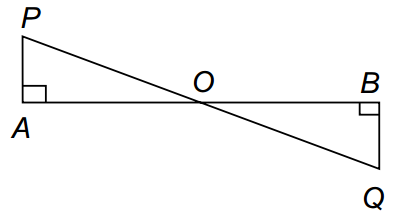A. PA = OB

B. AP = QB

C. OP = BQ

D. OA = OQ

Solution:

B

Example 4. In the given figure, triangles ABC and DCB are right angled at A and D respectively and AC = DB, then $$\Delta ABC\cong \Delta DCB$$ by ­__________ criterion.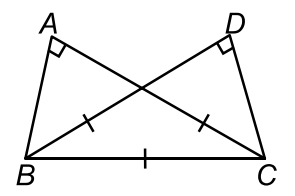A. AAA

B. SAS

C. ASS

D. None of these

Solution:

D

Example 5. In the given figure, ABC is an isosceles triangle in which AB = AC. If E and F be the midpoints of AC and AB respectively, then BE is equal to __________.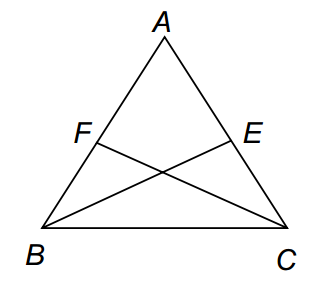A. CF

B. AB

C. CE

D. BF

Solution:

A: Since, AB = AC.

$$\frac{1}{2}AB=\frac{1}{2}AC\Rightarrow BF=EC$$.

Also,  $$AB=AC\Rightarrow \angle B=\angle C$$

(Angles opposite to equal sides are equal)

In  $$\Delta BEC$$ and  $$\Delta CFB$$:

EC = FB

$$\angle B=\angle C$$

BC = BC

$$\Delta BEC\cong \Delta CFB$$ (By SAS)

$$\Rightarrow BE=CF$$ (By CPCT)

Example 6. If you want to prove that $$\Delta FED\cong \Delta RQP$$ using the SAS criterion where  $$\widehat{D}=\widehat{P}$$, then which of the following you need more?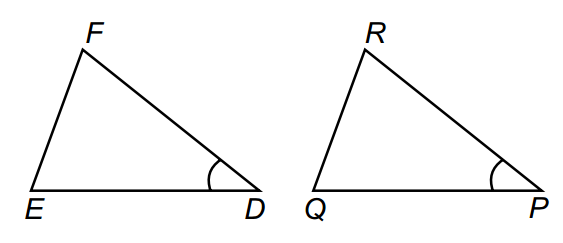A. FD = QP

B. QP = ED

C. FD = RP

D. Both (B) and (C)

Solution:

D: In  $$\Delta FED$$ and  $$\Delta RQP$$.

For SAS criterion, we must have

FD = RP

$$\angle D=\angle P$$

ED = QP

Then,  $$\Delta FED\cong \Delta RQP$$ (by SAS)

Example 7. Which congruence criterion can be used to conclude $$\Delta XYZ\cong \Delta PQR$$?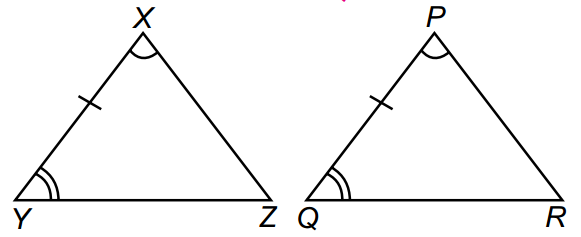A. SAS

B. SSS

C. RHS

D. None of these

Solution:

D: In  $$\Delta XYZ$$ and  $$\Delta PQR$$

$$\angle YXZ=\angle QPR$$ (given)

$$XY=PQ$$ (given)

$$\angle XYZ=\angle PQR$$ (given)

$$\therefore \text{ }\Delta XYZ\cong \Delta PQR$$ (By ASA)

Example 8. If $$\Delta AOC\cong \Delta BOD$$, then measure of  $$\widehat{OBD}$$ is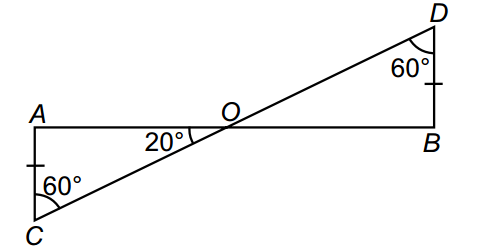A. 100O

B. 50O

C. 80O

D. None of these

Solution:

A: In  $$\Delta OAC$$

$$\angle OAC+\angle OCA+\angle AOC={{180}^{O}}$$ (angle sum property)

$$\angle OAC+{{60}^{O}}+{{20}^{O}}={{180}^{O}}\Leftrightarrow \angle OAC={{180}^{O}}-({{60}^{O}}+{{20}^{O}})={{100}^{O}}$$.

As  $$\Delta AOC\cong \Delta BOD$$

$$\therefore \text{ }\angle OAC=\angle OBD$$ (By CPCT)

So,  $$\angle OBD={{100}^{O}}$$.

Example 9. If $$\Delta ABC\cong \Delta PRQ$$, then  $$\widehat{B}$$ and PQ are respectively equal to

A. $$\widehat{P}$$ and AC

B.  $$\widehat{R}$$ and BC

C.  $$\widehat{R}$$ and AC

D.  $$\widehat{Q}$$ and AB

Solution:

C: Since,  $$\Delta ABC\cong \Delta PRQ$$.

$$\therefore$$ Their corresponding parts are equal.

$$\therefore \angle B=\angle R$$ and PQ = AC.

Example 10. In the given figure, if AB = AD and CB = CD, then which of the following is correct?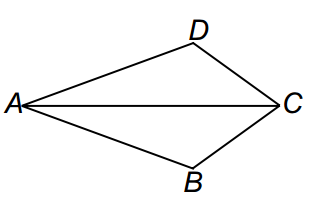A. $$\Delta ABC\cong \Delta ADC$$

B.  $$\widehat{BCA}=\widehat{DCA}$$

C.  $$\widehat{ADC}=\widehat{ABC}$$

D. All of these.

Solution:

D: In  $$\Delta ABC$$ and  $$\Delta ADC$$, we have

CB = CD (given)

AC = AC (common)

$$\therefore \text{ }\Delta ABC\cong \Delta ADC$$ (By SSS)

$$\therefore \text{ }\angle ADC=\angle ABC$$ and  $$\angle BCA=\angle DCA$$ (By CPCT)

Example 11. In the given figure, if $$\Delta OAP\cong \Delta OBQ$$, then which of the following is NOT true?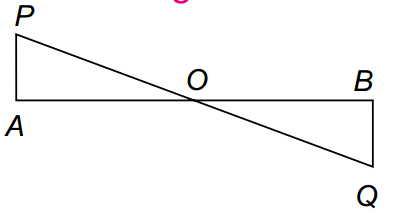A. AO = BO

B. AP = BQ

C. PO = BO

D.  $$\angle APO=\angle BQO$$.

Solution:

C: We have  $$\Delta OAP\cong \Delta OBQ$$, so , AP = BQ, AO = BO, OP = OQ and  $$\angle APO=\angle BQO$$,  $$\angle OAP=\angle OBQ$$,  $$\angle AOP=\angle BOQ$$.

Example 12. $$\Delta PQR\cong \Delta XYZ$$ by ________ congruency rule.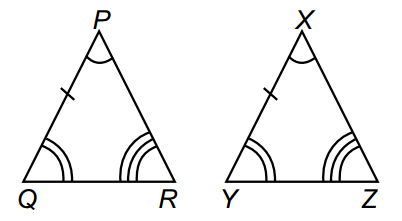A. SSS

B. AAA

C. SAS

D. ASA

Solution:

D: In  $$\Delta PQR$$ and  $$\Delta XYZ$$

$$\angle QPR=\angle YXZ$$ (given)

PQ = XY (given)

$$\angle PQR=\angle XYZ$$ (given)

$$\therefore \text{ }\Delta PQR\cong \Delta XYZ$$ (By ASA)

Example 13. Which congruence criterion can be used to state that $$\Delta XOY\cong \Delta POQ$$?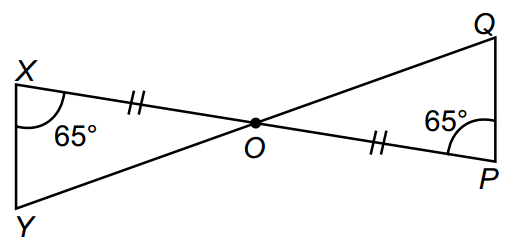A. ASA

B. SAS

C. SSS

D. RHS

Solution:

A: In  $$\Delta XOY$$ and  $$\Delta POQ$$.

$$\angle YXO=\angle QPO={{65}^{O}}$$ (given)

OX = OP (given)

And  $$\angle XOY=\angle POQ$$ (vertically opposite angles)

$$\therefore \text{ }\Delta XOY\cong \Delta POQ$$ (By ASA)

Direction (14 – 15): Study the figure and information given below carefully and answer the following questions.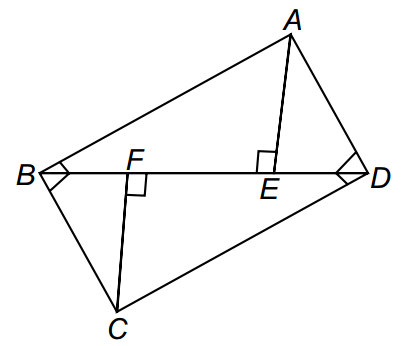CF and AE are equal perpendiculars on BD and BF = FE = ED.

Example 14. $$\Delta ABE$$ is congruent to

A. $$\Delta AED$$

B.  $$\Delta BFC$$

C.  $$\Delta CDF$$

D.  $$\Delta BCD$$

Solution:

C: In  $$\Delta ABE$$ and  $$\Delta CDF$$

AE = CF (given)

$$\angle AEB=\angle CFD={{90}^{O}}$$ (given)

BF + FE = DE + EF

$$\Rightarrow BE=DF\Rightarrow \Delta ABE\cong \Delta CDF$$ (By SAS)

Example 15.

$$\angle BAE=…………$$

A. $$\angle BCD$$

B.  $$\angle CBA$$

C.  $$\angle ADC$$

D.  $$\angle DCF$$

Solution:

D: As,  $$\Delta ABE\cong CDF$$

$$\therefore \text{ }\angle BAE=\angle DCF$$ (By CPCT)

Example 16. Select the odd one out.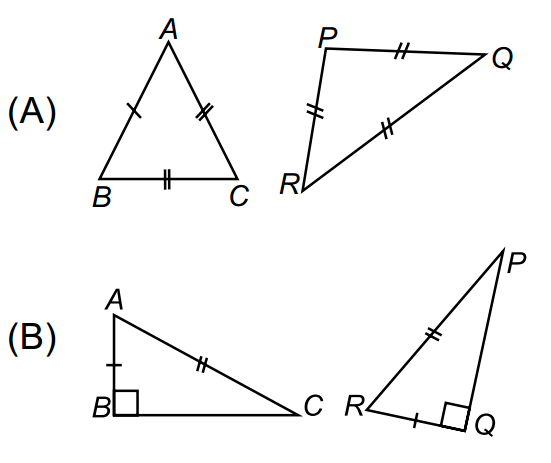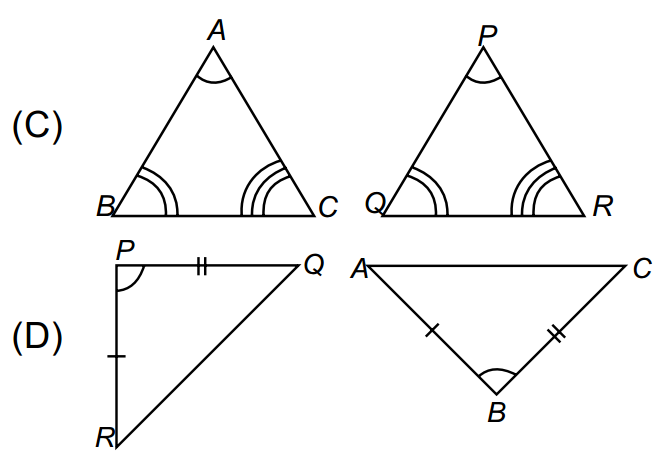Solution:

A: In all other options, triangles are congruent.

Example 17. If for $$\Delta ABC$$ and  $$\Delta DEF$$, the correspondence  $$CAB\leftrightarrow EDF$$ gives a congruence, then which of the following is NOT true?

A. AC = DE

B. AB = EF

C.  $$\angle A=\angle D$$

D.  $$\angle C=\angle E$$

Solution:

B: We can conclude that CA = ED, AB = DF, CB = EF,  $$\angle CAB=\angle EDF$$,  $$\angle ACB=\angle DEF$$,  $$\angle ABC=\angle DFE$$.

Example 18. By which congruency criterion, $$\Delta PQR\cong \Delta PQS$$?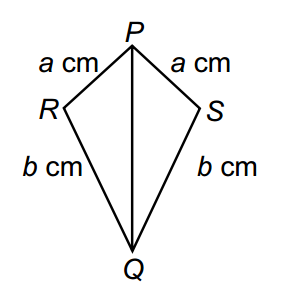A. RHS

B. ASA

C. SSS

D. SAS

Solution:

C: In  $$\Delta PQR$$ and  $$\Delta PQS$$

PR = PS = a cm (given)

RQ = SQ = b cm (given)

PQ = PQ  (common)

$$\therefore \text{ }\Delta PQR\cong \Delta PQS$$ (By SSS)

Example 19. In two triangles PQR and LMN, PQ = QR, $$\angle P=\angle M$$ and QR = LN, then which of the following statements is CORRECT?

A. Triangles are congruent only.

B. Triangles are isosceles only.

C. Triangles are both congruent and isosceles.

D. None of these.

Solution:

D

Example 20. In the given figure, if PR = QR, $$\angle SRP=\angle TRQ$$ and  $$\angle SQP=\angle TPQ$$, then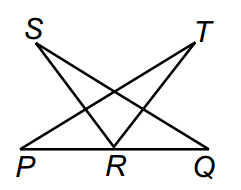A. $$\Delta SQR\cong \Delta PTR\And SR=TR$$

B.  $$\Delta SQR\cong \Delta TPR\And SR=TR$$

C. $$\Delta RQS\cong \Delta TPR\And SR=TR$$

D.  $$\Delta QRS\cong \Delta PTR\And SR=PT$$

Solution:

B